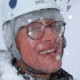## Le Monde puzzle [#5]

Another Sudoku-like puzzle from the weekend edition of Le Monde. The object it starts with is a 9×9 table where each entry is an integer and where neighbours take adjacent values. (Neighbours are defined as north, west, south and east of an entry.) The question is about whether or not it is possible to find a table such that the sum of the 81 entries is 99. The problem is equivariant under location change, namely if each entry is shifted by a, the constraints are still satisfied but the sum is modified by 81a. If, for instance, a is the (5,5) entry, the overall sum is 81a plus an even number between -360 and +360. Looking at the generation of such tables, I wrote the simple-minded and lengthy  R code that follows:

```#Generating an acceptable grid
#where neighbours in space must be neighbours in value

grid=matrix(0,9,9)
neigh=grid*0
#start from the centre
neigh[5,5]=1

for (t in 1:8){

neigh[neigh==1]=2

for (i in 1:9){
for (j in 1:9){

if (neigh[i,j]==0){

vois=NULL
if ((j>1)&&(neigh[i,j-(j>1)]==2)){vois=rbind(vois,c(i,j-1))}
if ((j<9)&&(neigh[i,j+(j<9)]==2)){vois=rbind(vois,c(i,j+1))}
if ((i<9)&&(neigh[i+(i<9),j]==2)){vois=rbind(vois,c(i+1,j))}  if ((j>1)&&(neigh[i-(i>1),j]==2)){vois=rbind(vois,c(i-1,j))}

neigh[i,j]=1-is.null(vois)
}

if (neigh[i,j]==1){

if (dim(vois)==1){

grid[i,j]=grid[vois]+sample(c(-1,1),1)}else{

if (min(grid[vois])!=max(grid[vois])){
grid[i,j]=round(mean(grid[vois]))}else{
grid[i,j]=grid[vois[1,1],vois[1,2]]+sample(c(-1,1),1)}
}
}}
}}
```

which provides a random grid centred at zero and satisfying the assumptions. Here is one instance for grid

```> grid
[,1] [,2] [,3] [,4] [,5] [,6] [,7] [,8] [,9]
[1,]    1    0    1    2    1    0   -1    0   -1
[2,]    0   -1    0    1    0   -1    0    1    0
[3,]    1    0   -1    0    1    0    1    2    1
[4,]    2    1    0    1    2    1    2    1    0
[5,]    1    2    1    0    1    0    1    2    1
[6,]    2    1    0   -1    0    1    0    1    2
[7,]   -1    0   -1    0   -1    0   -1    0    1
[8,]    0    1    0    1    0    1    0   -1    0
[9,]    1    0   -1    0   -1    0   -1    0    1
```

A few thousand simulations show that achieving 99[-81] or 99[+81] (i.e. when centred at 1 or -1) is indeed possible. Here is a grid leading to a total sum of 99:

```       [,1] [,2] [,3] [,4] [,5] [,6] [,7] [,8] [,9]
[1,]    1    0   -1    0    1    0    1    2    3
[2,]    2    1    0   -1    0    1    2    1    2
[3,]    3    2    1    0    1    2    3    2    3
[4,]    4    3    2    1    2    3    2    1    2
[5,]    3    2    1    2    1    2    1    0    1
[6,]    0    1    2    1    0    1    0    1    0
[7,]    1    0    1    2    1    2    1    0    1
[8,]    0   -1    0    1    2    3    2    1    0
[9,]   -1    0    1    2    1    2    3    2    1
```

### 5 Responses to “Le Monde puzzle [#5]”

1.Le Monde puzzle [#6] « Xi'an's Og Says:

[…] simple challenge in Le Monde this week: find the group of four primes such that any sum of three terms in the group is prime and […]

2.xi'an Says:

I have just received the next issue of Le Monde and, lo and behold!, they have restricted themselves to non-negative integers… Not very interesting and lacking mathematical structure, in my humble opinion!

3.Robin Ryder Says:

Actually, any sum is possible. Paint the grid like a chessboard, with 41 black squares and 40 white squares. Put a 1 in all the black squares. Each white square can take the values 0 or 2, leading to all odd sums between 41+40×0=41 and 41+40×2=121 (in particular, using 24 2’s and 16 0’s gives 99). Similarly, putting 1’s in the white squares leads to all even numbers between 40 and 122. As you mentioned, you can get any other sum by adding a multiple of 81.

•xi'an Says:

Sure, I completely agree. My reasoning was based on sums obtained from a central (5,5) entry equal to zero. But your black&white argument is much cleaner and much quicker!

•Peter Green Says:

Err… 41+24*2=89. You mean 29 2’s. But it’s a nice way to think about the problem. Note that it also proves (e.g.) there is no solution with an even number in the middle of the grid.

This site uses Akismet to reduce spam. Learn how your comment data is processed.